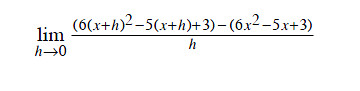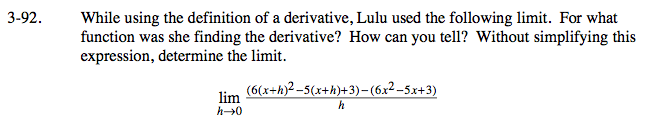### Home > CALC > Chapter 3 > Lesson 3.3.1 > Problem3-92

3-92.
1. While using the definition of a derivative, Lulu used the following limit. For what function was she finding the derivative? How can you tell? Without simplifying this expression, determine the limit. Homework Help ✎Lulu used Hana's definition of the derivative;

$f'(x)=\lim_{h\rightarrow 0}\frac{f(x+h)-f(x)}{h}$

Examine Lulu's limit: What if f(x)?

Once you determine f(x), use the Power Rule to find f '(x), the derivate function.This derivative function is the value of Lulu's limit as h→0.